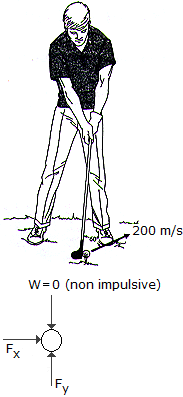# Engineering Mechanics - KOP: Impulse and Momentum - Discussion

### Discussion :: KOP: Impulse and Momentum - General Questions (Q.No.1)

1.A golf ball having a mass of 40 g is struck such that it has an initial velocity of 200 m/s as shown. Determine the horizontal and vertical components of the impulse given to the ball.

 [A]. Impx = 4.00 N-s, Impy = 6.93 N-s [B]. Impx = 6.93 N-s, Impy = 4.00 N-s [C]. Impx = 6.93 N-s, Impy = 4.39 N-s [D]. Impx = 4.00 N-s, Impy = 7.32 N-s

Explanation:

No answer description available for this question.

 Dhananjay said: (Sep 16, 2011) Please give the solution.

 Mithun said: (Dec 2, 2015) Impulse = Force*Time.

 Rameez said: (Apr 8, 2016) I need answer and explanation.

 Kavya said: (Nov 23, 2016) Please give the solution.

 Aakanksha Patekar said: (Mar 2, 2017) Please explain the answer with correct explanation.

 Srikanth said: (Aug 23, 2019) Please explain it in detail.

 Nathan said: (Jan 19, 2021) Components of impulse Since mu=impulse then let's find the components of u. The angle is 90-60=30. Ux=200cos30, =173.2m/s. Components of Uy=200sin30. =100m/s. So the impulses respectively is 173.2*0.04. = 6.928Ns. And the vertical is 100*0.04, =4Ns.